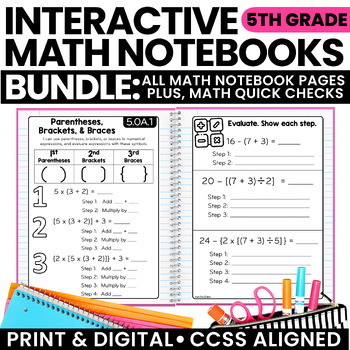# Math Interactive Notebook 5th Grade BUNDLE | Digital & Printable | Google Slides4th - 6th, Homeschool
Subjects
Standards
Resource Type
Formats Included
• Zip
Pages
180+
\$21.50
Bundle
List Price:
\$25.75
You Save:
\$4.25
\$21.50
Bundle
List Price:
\$25.75
You Save:
\$4.25
##### Also included in
1. This BUNDLE includes everything you need for 5th grade math!!★ Interactive Notebook Pages★ Math Quick Checks ★ Math Menus (Perfect for homework or early finishers!!)★ Open-Ended Math Pages★ Math Exit Slips★ Math Mastery Task Cards★ Printables for DecimalsFeel free to email with any questions or comm
\$57.50
\$70.70
Save \$13.20

### Description

What are Interactive Math Notebooks?

Interactive math notebooks are teaching pages used to introduce and teach math concepts and skills. Each set of notebook pages includes definitions, explanations, rules, and algorithms that may be needed to address each standard. Practice problems are included to be used for guided practice. Once completed, these pages serve as a great reference tool for students to refer back throughout the year.

How do I use them with my students?

Notebook pages can be used to introduce, reinforce, or review math standards. They can be used for direct instruction for a whole class or in small groups. For the printable version, students first cut out the pages and glue them into a spiral notebook. With teacher guidance, students take notes and work out practice problems. Students can then highlight or add color to their notebook pages. The digital pages include the same content, but require no cutting or pasting!

What materials are needed for printable notebook pages?

A spiral notebook for each student, scissors, glue sticks, pencils, and colored pencils, crayons, markers (optional)

What is included in this BUNDLE?

• Notebook pages for each of the 4th grade math standards. Each concept or skill includes a double-page spread. (Printable & Digital)
• Answer Key (Available with printable pages)
• Math Quick Checks for all 4th grade math standards

(For a more detailed PREVIEW, please preview each of the individual products above!)

★★This resources in this bundle are also included in my ULTIMATE 5th Grade Math Bundle!!★★

Check out the rest of my Math Activities for 5th Grade:

5th Grade Interactive Math Notebook: OA & NBT

5th Grade Interactive Math Notebook: NF

5th Grade Interactive Math Notebook: MD & G

Math Quick Checks

Math Exit Slips

Total Pages
180+
Included
Teaching Duration
1 Year
Report this Resource to TpT
Reported resources will be reviewed by our team. Report this resource to let us know if this resource violates TpT’s content guidelines.

### Standards

to see state-specific standards (only available in the US).
Use parentheses, brackets, or braces in numerical expressions, and evaluate expressions with these symbols.
Write simple expressions that record calculations with numbers, and interpret numerical expressions without evaluating them. For example, express the calculation âadd 8 and 7, then multiply by 2â as 2 Ã (8 + 7). Recognize that 3 Ã (18932 + 921) is three times as large as 18932 + 921, without having to calculate the indicated sum or product.
Generate two numerical patterns using two given rules. Identify apparent relationships between corresponding terms. Form ordered pairs consisting of corresponding terms from the two patterns, and graph the ordered pairs on a coordinate plane. For example, given the rule âAdd 3â and the starting number 0, and given the rule âAdd 6â and the starting number 0, generate terms in the resulting sequences, and observe that the terms in one sequence are twice the corresponding terms in the other sequence. Explain informally why this is so.
Add and subtract fractions with unlike denominators (including mixed numbers) by replacing given fractions with equivalent fractions in such a way as to produce an equivalent sum or difference of fractions with like denominators. For example, 2/3 + 5/4 = 8/12 + 15/12 = 23/12. (In general, ð¢/ð£ + ð¤/ð¥ = (ð¢ð¥ + ð£ð¤)/ð£ð¥.)
Solve word problems involving addition and subtraction of fractions referring to the same whole, including cases of unlike denominators, e.g., by using visual fraction models or equations to represent the problem. Use benchmark fractions and number sense of fractions to estimate mentally and assess the reasonableness of answers. For example, recognize an incorrect result 2/5 + 1/2 = 3/7, by observing that 3/7 < 1/2.